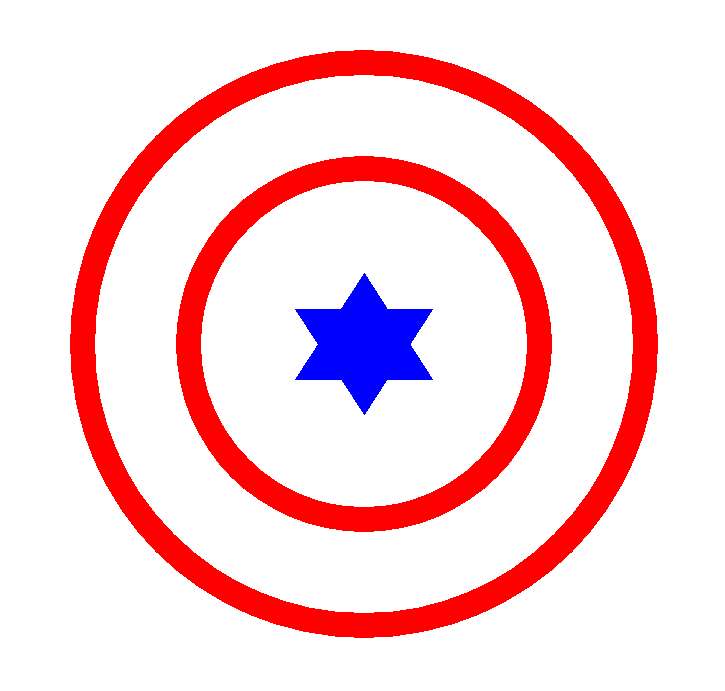/ Visualization

# Drawing Capitan America Logo!

This post is motivated by this post on KANDINSKY (http://giorasimchoni.com/2017/07/30/2017-07-30-data-paintings-the-kandinsky-package/)``````
library(grid)
grid.newpage()

grid.circle(x = 0.5, y = 0.5, r = 0.4,
gp = gpar(lwd = 25, col = "red", fill = "white"))

grid.circle(x = 0.5, y = 0.5, r = 0.25,
gp = gpar(lwd = 25, col = "red", fill = "white"))

x= c(0.45, 0.50, 0.55)
y= c(0.45, 0.60, 0.45)
grid.polygon(x=x, y=y, gp=gpar(fill="blue", col="blue")) # outer shell

x= c(0.45, 0.50, 0.55)
y= c(0.55, 0.40, 0.55)
grid.polygon(x=x, y=y, gp=gpar(fill="blue", col="blue")) # outer shell
``````